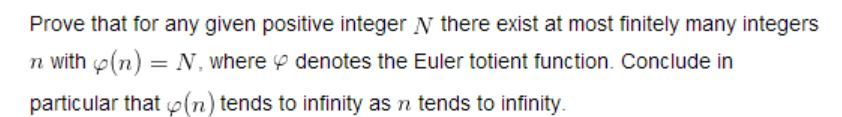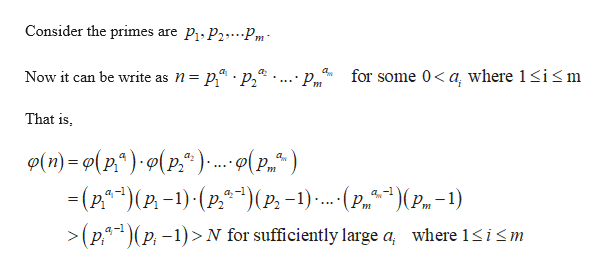# Prove that for any given positive integer N there exist at most finitely many integersdenotes the Euler totient function. Conclude inn with(n)N, whereparticular that (n) tends to infinity as n tends to infinity.

Question
17 views

Question attached.  I have found solutions to this probem on the internet, but I don't understand the explanations.  Can you provide a "layman's terms" explanation?  Thanks.help_outlineImage TranscriptioncloseProve that for any given positive integer N there exist at most finitely many integers denotes the Euler totient function. Conclude in n with(n) N, where particular that (n) tends to infinity as n tends to infinity. fullscreen
check_circle

Step 1

Let N be a given positive integer and let p be the least prime number greater than N + 1.

Let n be an integer such that

Step 2

If q > p is a prime divisor of n, then n = (qk) m for some k > 1and m with q not diving m.

Step 3

It is contraction to our assumption.

Thus, there is no prime divisor of n is greater than N + 1.

In p...help_outlineImage TranscriptioncloseP1 P2 P Consider the primes are m. for some 0 a, where 1sim a Pm Now it can be write as n = P^" - P2 That is Ф(m) -о(p*) -о(p:*). o(p.*) - (2**) (P-1)-(р.*)(P.-1). . (р, з)(р. - _ )(Pm-1) iśm a-l >(p1P-1)N for sufficiently large a where 1 fullscreen

### Want to see the full answer?

See Solution

#### Want to see this answer and more?

Solutions are written by subject experts who are available 24/7. Questions are typically answered within 1 hour.*

See Solution
*Response times may vary by subject and question.
Tagged in

### Math